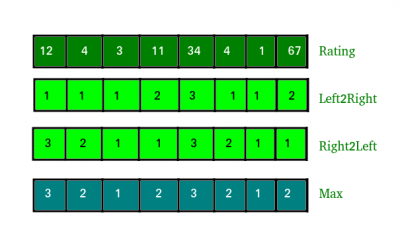# Find minimum cost to buy all books

• Difficulty Level : Medium
• Last Updated : 22 Jun, 2021

Given an array of ratings for n books. Find the minimum cost to buy all books with below conditions :

1. Cost of every book would be at-least 1 dollar.
2. A book has higher cost than an adjacent (left or right) if rating is more than the adjacent.

Examples :

```Input : Ratings[] = {1, 3, 4, 3, 7, 1}
Output : 10
Exp :- 1 + 2 + 3 + 1 + 2 + 1 = 10

Input : ratings[] = {1, 6, 8, 3, 4, 1, 5, 7}
Output : 15
Exp :- 1 + 2 + 3 + 1 + 2 + 1 + 2 + 3 = 15 ```

1. Make two arrays left2right and right2left and fill 1 in both of them.
2. Traverse left to right and fill left2right array and update it by seeing previous rating of given array. Do not care about next rating of given array.
3. Traverse right to left and fill right2left array and update it by seeing next rating of given array. Do not care about previous rating of given array.
4. Find maximum value of ith position in both array (left2right and right2left) and add it to result## C++

 `// C++ program to find minimum cost to buy``// n books.``#include ``using` `namespace` `std;` `int` `minCost(``int` `ratings[], ``int` `n)``{``    ``int` `res = 0;``    ``int` `left2right[n];``    ``int` `right2left[n];` `    ``// fill 1 in both array``    ``fill_n(left2right, n, 1);``    ``fill_n(right2left, n, 1);` `    ``// Traverse from left to right and assign``    ``// minimum possible rating considering only``    ``// left adjacent``    ``for` `(``int` `i = 1; i < n; i++)``        ``if` `(ratings[i] > ratings[i - 1])``            ``left2right[i] = left2right[i - 1] + 1;    ` `    ``// Traverse from right to left and assign``    ``// minimum possible rating considering only``    ``// right adjacent``    ``for` `(``int` `i = n - 2; i >= 0; i--)``        ``if` `(ratings[i] > ratings[i + 1])``            ``right2left[i] = right2left[i + 1] + 1;      ``    ` `    ``// Since we need to follow rating rule for``    ``// both adjacent, we pick maximum of two``    ``for` `(``int` `i = 0; i < n; i++)``        ``res += max(left2right[i], right2left[i]);``    ` `    ``return` `res;``}` `// Driver function``int` `main()``{``    ``int` `ratings[] = { 1, 6, 8, 3, 4, 1, 5, 7 };``    ``int` `n = ``sizeof``(ratings) / ``sizeof``(ratings);``    ``cout << minCost(ratings, n);``    ``return` `0;``}`

## Java

 `// JAVA Code For Find minimum cost to``// buy all books``import` `java.util.*;` `class` `GFG {``    ` `    ``public` `static` `int` `minCost(``int` `ratings[], ``int` `n)``    ``{``        ``int` `res = ``0``;``        ``int` `left2right[] = ``new` `int``[n];``        ``int` `right2left[] = ``new` `int``[n];;``     ` `        ``// fill 1 in both array``        ``Arrays.fill(left2right,  ``1``);``        ``Arrays.fill(right2left, ``1``);``     ` `        ``// Traverse from left to right and assign``        ``// minimum possible rating considering``        ``// only left adjacent``        ``for` `(``int` `i = ``1``; i < n; i++)``            ``if` `(ratings[i] > ratings[i - ``1``])``                ``left2right[i] = left2right[i - ``1``] + ``1``;    ``     ` `        ``// Traverse from right to left and assign``        ``// minimum possible rating considering only``        ``// right adjacent``        ``for` `(``int` `i = n - ``2``; i >= ``0``; i--)``            ``if` `(ratings[i] > ratings[i + ``1``])``                ``right2left[i] = right2left[i + ``1``] + ``1``;      ``         ` `        ``// Since we need to follow rating rule for``        ``// both adjacent, we pick maximum of two``        ``for` `(``int` `i = ``0``; i < n; i++)``            ``res += Math.max(left2right[i],``                            ``right2left[i]);``         ` `        ``return` `res;``    ``}``    ` `    ``/* Driver program to test above function */``    ``public` `static` `void` `main(String[] args)``    ``{``        ``int` `ratings[] = { ``1``, ``6``, ``8``, ``3``, ``4``, ``1``, ``5``, ``7` `};``        ``int` `n = ratings.length;``        ``System.out.print(minCost(ratings, n));``        ` `    ``}``}``  ` `// This code is contributed by Arnav Kr. Mandal.`

## Python

 `# Python program to find minimum cost to buy``# n books.` `def` `minCost(ratings, n):``    ``res ``=` `0``   ` `    ``# fill 1 in both array``    ``left2right ``=` `[``1` `for` `i ``in` `range``(n)]``    ``right2left ``=` `[``1` `for` `i ``in` `range``(n)]`` ` `    ``# Traverse from left to right and assign``    ``# minimum possible rating considering only``    ``# left adjacent``    ``for` `i ``in` `range``(``1``, n):``        ``if` `(ratings[i] > ratings[i ``-` `1``]):``            ``left2right[i] ``=` `left2right[i ``-` `1``] ``+` `1`` ` `    ``# Traverse from right to left and assign``    ``# minimum possible rating considering only``    ``# right adjacent``    ``i ``=` `n ``-` `2``    ``while``(i >``=` `0``):``        ``if` `(ratings[i] > ratings[i ``+` `1``]):``            ``right2left[i] ``=` `right2left[i ``+` `1``] ``+` `1``        ``i ``-``=` `1``     ` `    ``# Since we need to follow rating rule for``    ``# both adjacent, we pick maximum of two``    ``for` `i ``in` `range``(n):``        ` `        ``res ``+``=` `max``(left2right[i], right2left[i])``     ` `    ``return` `res`` ` `# Driver function``ratings ``=` `[ ``1``, ``6``, ``8``, ``3``, ``4``, ``1``, ``5``, ``7` `]``n ``=` `len``(ratings)``print` `minCost(ratings, n)` `# This code is contributed by Sachin Bisht`

## C#

 `// C# code For Finding minimum``// cost to buy all books``using` `System;` `class` `GFG {``    ` `    ``public` `static` `int` `minCost(``int` `[]ratings, ``int` `n)``    ``{``        ``int` `res = 0;``        ``int` `[]left2right = ``new` `int``[n];``        ``int` `[]right2left = ``new` `int``[n];``    ` `        ``// fill 1 in both array``        ``for``(``int` `i = 0; i < n; i++)``        ``left2right[i] = 1;``        ` `        ``for``(``int` `i = 0; i < n; i++)``        ``right2left[i] = 1;``    ` `        ``// Traverse from left to right and assign``        ``// minimum possible rating considering``        ``// only left adjacent``        ``for` `(``int` `i = 1; i < n; i++)``            ``if` `(ratings[i] > ratings[i - 1])``                ``left2right[i] = left2right[i - 1] + 1;    ``    ` `        ``// Traverse from right to left and assign``        ``// minimum possible rating considering only``        ``// right adjacent``        ``for` `(``int` `i = n - 2; i >= 0; i--)``            ``if` `(ratings[i] > ratings[i + 1])``                ``right2left[i] = right2left[i + 1] + 1;    ``        ` `        ``// Since we need to follow rating rule for``        ``// both adjacent, we pick maximum of two``        ``for` `(``int` `i = 0; i < n; i++)``            ``res += Math.Max(left2right[i],``                            ``right2left[i]);``        ` `        ``return` `res;``    ``}``    ` `    ``/* Driver program to test above function */``    ``public` `static` `void` `Main()``    ``{``        ``int` `[]ratings = {1, 6, 8, 3, 4, 1, 5, 7};``        ``int` `n = ratings.Length;``        ``Console.Write(minCost(ratings, n));``    ``}``}``    ` `// This code is contributed by nitin mittal.`

## Javascript

 ``

Output:

`15`

Time complexity – O(n)
Auxiliary Space – O(n)
This article is contributed by Harshit Agrawal. If you like GeeksforGeeks and would like to contribute, you can also write an article using write.geeksforgeeks.org or mail your article to review-team@geeksforgeeks.org. See your article appearing on the GeeksforGeeks main page and help other Geeks.